### Home > CALC > Chapter Ch2 > Lesson 2.2.2 > Problem2-72

2-72.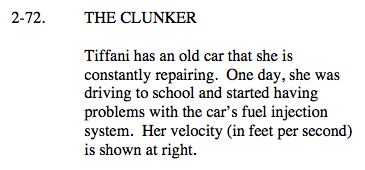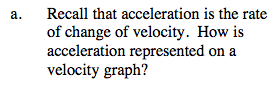$\text{acceleration }=\text{ rate of change of velocity }= \frac{\frac{\text{feet}}{\text{second}}}{\text{second}}.$

Notice what each axis represents.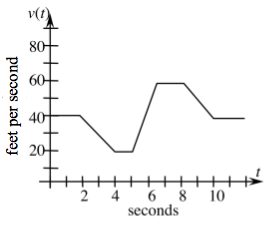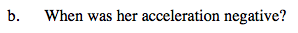negative acceleration = decreasing velocity. The first time this happens is between t = 2 and t = 4. When else does this happen?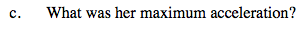On what interval is Tiffani's velocity increasing the most? Remember, this is a velocity graph.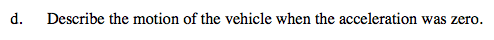0 acceleration means that velocity is not changing. It does NOT mean that velocity is 0. What does it mean then? Notice that Tiffani was moving with 0 acceleration during three intervals on her journey.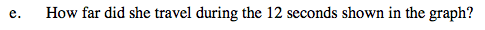Displacement can be determined by calculating the area under a velocity curve.